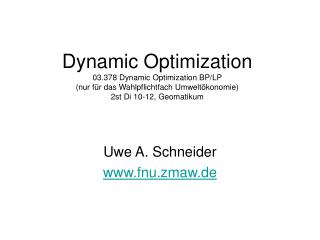DownloadDownload PresentationDynamic Optimization 03.378 Dynamic Optimization BP/LP (nur für das Wahlpflichtfach Umweltökonomie) 2st Di 10-12, Geoma

# Dynamic Optimization 03.378 Dynamic Optimization BP/LP (nur für das Wahlpflichtfach Umweltökonomie) 2st Di 10-12, Geoma

Download Presentation## Dynamic Optimization 03.378 Dynamic Optimization BP/LP (nur für das Wahlpflichtfach Umweltökonomie) 2st Di 10-12, Geoma

- - - - - - - - - - - - - - - - - - - - - - - - - - - E N D - - - - - - - - - - - - - - - - - - - - - - - - - - -
##### Presentation Transcript

1. Dynamic Optimization03.378 Dynamic Optimization BP/LP(nur für das Wahlpflichtfach Umweltökonomie) 2st Di 10-12, Geomatikum Uwe A. Schneider www.fnu.zmaw.de

2. Topics • Review Integration, Simple Differential Equations • Calculus of Variation • Optimal Control Theory • Phase Diagrams / Stability Analysis • Dynamic Programming

3. Integration and Dynamic Optimization • Integration often needed for solving systems of differential equations • Euler's Equation of COV • Hamiltonian of Optimal Control • more difficult than differentiation • computers and look-up tables make life easier

4. Standard Integrals

5. Standard Integrals

6. Simple Integration Rules • Sums: • Powers: • Constant coefficients: • Log: • Exponentials:

7. Variable Separation • Derived from chain rule of differentiation

8. Integration by Parts • Derived from product rule of differentiation

9. Integration by Substitution • Derived from chain rule of differentiation

10. Integrating Factor • A function by which an ordinary differential equation is multiplied in order to make it integrable • Used to aid solving • linear first and higher order differential equations • bernoulli equations • non-exact equations • several others • Makes a differential equation look like a known antiderivative • A given differential equation may have zero, 1, or more integrating factors

11. Differential Equations, 1 • Equations that involve dependent variables and their derivatives with respect to the independent variables are called differential equations • Ordinary Differential Equation: Differential equations that involve only ONE independent variable

12. Differential Equations, 2 • Order: The order of a differential equation is the highest derivative that appears in the differential equation • Degree: The degree of a differential equation is the power of the highest derivative term. … Second order of degree 3

13. Differential Equations, 2 • Linear … if there are no multiplications among dependent variables and their derivatives … all coefficients are functions of independent variables • Non-linear … do not satisfy the linear condition • Quasi-linear … if there are no multiplications among all dependent variables and their derivatives in the highest derivative term

14. Differential Equations, 3 • Homogeneous … if every single term contains the dependent variables or their derivatives. • Non-homogeneous … Otherwise • Autonomous … if independent variable does not appear in the equation • Exact vs. nonexact (see later slides)

15. Linear First Order Differential Equations (FODE) • Constant coefficients: • Variable coefficients:

16. General Solution to Linear FODE Integrating Factor

17. Particular Solution to Linear FODE

18. General Solution to Linear FODE Integrating Factor

19. Linear Second Order Differential Equations (FODE) • Constant coefficients: • Variable coefficients:

20. Bernoulli Equation • Fundamental equation of motion for neo-classical growth models with Cobb-Douglas technology • Use integrating factor: (1-a)y-a • Substitute k by z = y(1-a) yielding: • Solve this linear first order differential equation • Substitute z by y = z1/(1-a)

21. Exact Equations, 1

22. Exact Equations, 2

23. Exact Equations, 3 • Integrate to find (y) • Note that (y) is a function of y only. Therefore, in the expression giving '(y) the variable, x, should disappear. • Write down the function F(x,y) • All solutions given by F(x,y) = k • Alternatively, one can integrate over y and use (x)

24. Conversion of Nonexact Equations

25. Calculus of Variation • Find path x(t), which optimizes • F(.) is twice differentiable • Starting and end points are known

26. Derivation of First Order Conditions • Decompose x(t) into optimal path x*(t) + deviation a*h(t) • Setup • Evaluate g’(0) = 0 (Optimum is where g' = 0 and a = 0)

27. First Order Necessary Condition = Euler Equation

28. Second Order Necessary Conditions = Legendre condition • Maximization: • Minimization:

29. Sufficient Conditions • F is jointly concave in x and x' for a maximization • F is jointly convex in x and x' for a minimization

30. Production and Inventory Planning Example • Need to deliver B units at T • Production cost rise linearly with production rate • Cost of holding inventory is constant per unit of time • Zero inventory at beginning • Minimize total cost

31. Production and Inventory Planning

32. Problem Extensions • Fixed starting point - free end value • Fixed starting point - free horizon • Transversality conditions • Salvage value • Several Functions

33. Free End Value

34. Free End Value

35. Free Starting Value, Solution Free Starting and End Value, Solution

36. Free Horizon

37. Solution to Free Horizon Problem

38. Terminal Function

39. Solution to Terminal Function Problem

40. Terminal Function with Salvage Value

41. Terminal Function with Salvage ValueSolution

42. Several Functions

43. Several Functions, Solution

44. Limitations • Functional constraints • Continuity • Differentiability • Integrability (to solve for x(t)) • Set-up • Continuous decisions • Simple functions

45. Optimal Control

46. Optimal Control (OC) • Generalization of Calculus of Variation • Pontryagin, L.S. et al. late 1950's • State variable(s) … x(t) • Control variable(s) … u(t) • can deal with corner points • can have binary variables (0,1)

47. OC – Simplest Problem Objective function } State equation Boundary Conditions

48. Relationship to COV • letting u(t) = x'(t) • yields calculus of variation problem with free end value